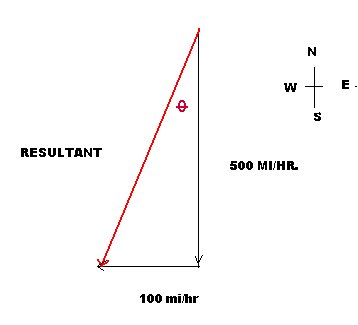# Difference Between Vectors and Scalars

The basic difference between a scalar and vector quantity can be identified by the parameters which define these quantities.

A scalar quantity is a number, which indicates the magnitude of anything under consideration – a medium. The vector, on the other hand, not only needs a magnitude but also direction. There are many applications of these quantities in science, especially physics.

In this article we will explain these two quantities with the help of appropriate examples.

### Instructions

• 1

Scalar

A scalar is a one dimensional description of any quantity, representing only its magnitude. It does not give any indication about the direction of motion or flow. The basic example of a scalar quantity is speed.

When we say that a car is running at a speed of 80 kilometers per hours, we don’t give any indication about the direction of motion.

Similarly, when an object is at 120 degree Fahrenheit, we only get a measure of the magnitude of hotness; it does not indicate the direction in which the heat of the object will flow.

Time is also a major example of scalar quantities. When we express the time in months, days, hours, minutes and seconds, it only indicates a quantity.

Similarly a volume of any object only gives the indication of the space occupied by it, and no description about the direction of motion.

Image courtesy: faculty.plattsburgh.edu• 2

Vector

Vector quantities need both magnitude and direction to be defined properly.

Considering the above example of speed, when a person moves at a certain speed, there is no direction involved, but when he/she moves with a velocity – rate of change of speed – then there is a direction attached, indicating whether the speed is low or high.

In order to be defined a vector quantity though; there are a certain pre requisites.

There should be an indication of direction, for example, forward, backward, towards left or right, or at an angle etc.

There should be an initial reference point, which can be used to determine the direction easily. This reference point should have its centre in either north, south, east or west quadrant so that the direction can be easily referenced.

Thus when we said that a car was moving at 80 kilometers per hours to define a scalar quantity, if we add 80 kilometers per hours towards north, it becomes a vector quantity.

Other examples of vector quantities are moments, forces and accelerations.

Image courtesy: edinformatics.com•